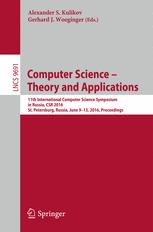• A
• A
• A
• АБB
• АБB
• АБB
• А
• А
• А
• А
• А
Обычная версия сайта

## Algorithmic Statistics: Normal Objects and Universal Models

P. 280-293.

In algorithmic statistics quality of a statistical hypothesis (a model) P for a data x is measured by two parameters: Kolmogorov complexity of the hypothesis and the probability P(x). A class of models SijSij that are the best at this point of view, were discovered. However these models are too abstract. To restrict the class of hypotheses for a data, Vereshchaginintroduced a notion of a strong model for it. An object is called normal if it can be explained by using strong models not worse than without this restriction. In this paper we show that there are “many types” of normal strings. Our second result states that there is a normal object x such that all models SijSij are not strong for x. Our last result states that every best fit strong model for a normal object is again a normal object.

### В книгеVol. 9691: Lecture Notes in Computer Science. Switzerland: Springer, 2016.